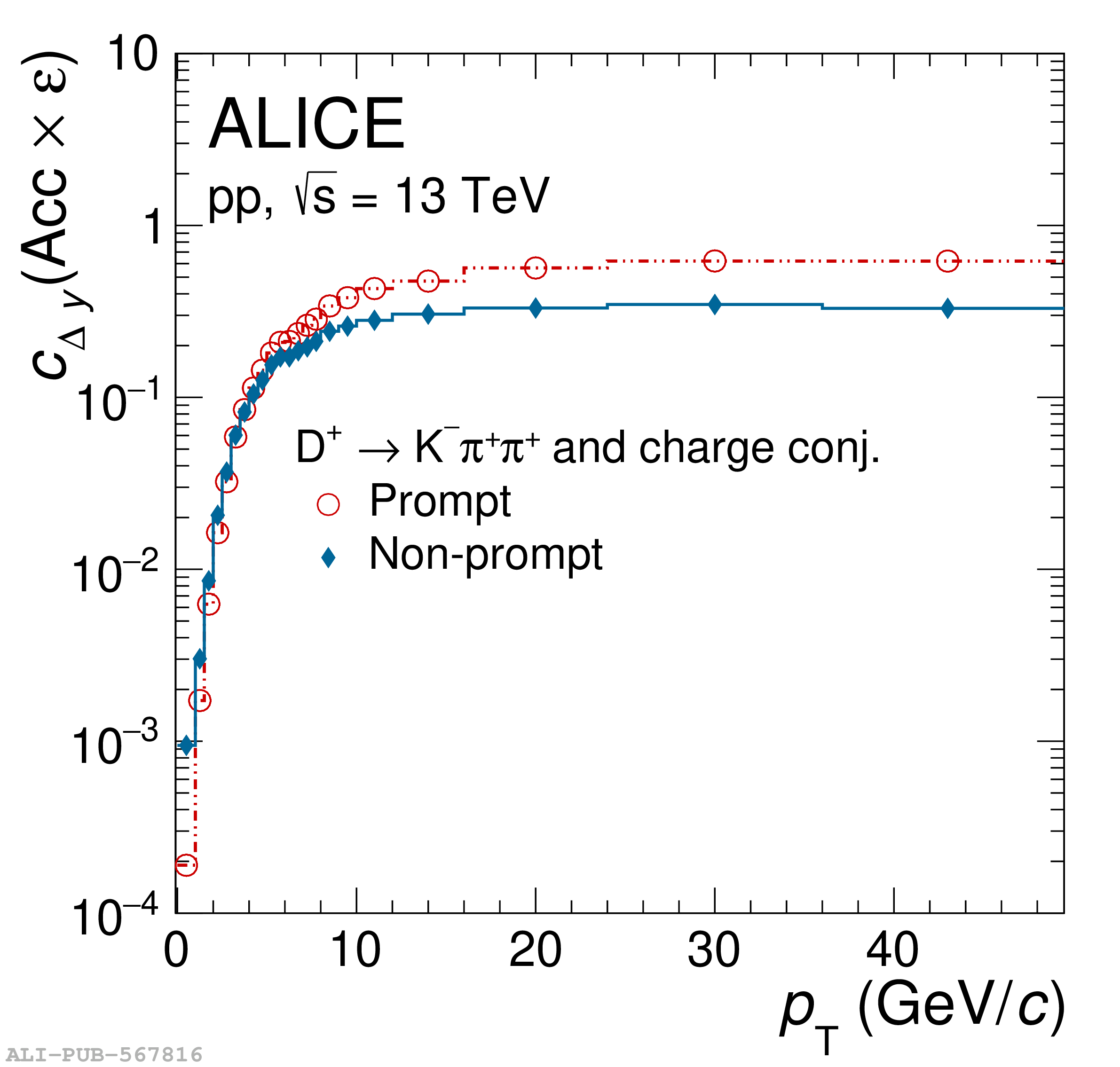# Figure 3

 Correction factors $c_{\Delta y}({\rm Acc}\times \varepsilon)$ for the prompt and non-prompt $\Dzero$-meson (top-left), the $\Dplus$-meson (top-right), the $\Dstar$-meson (bottom-left), and the $\Ds$-meson (bottom-right) as a function of $\pt$.